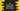# C# string Join method explanation with example## C# string Join method explanation with example:

Join method is used to join the elements of an array. We can join the strings of an array or any other objects of an array. With this method, we can also add one separator to the joined values. In this post, we will learn how to use Join with different examples.

### Syntax of Join:

The Join method is defined as below:

``````public static string Join(String s, params String[] arr)
public static string Join(String s, params Object[] arr)
public static string Join(String s, params IEnumerable<String> arr)``````

Here,

• s is the separator to use in the joined string.
• arr is the array.

### Example of Join:

Let’s take a look at the below example:

``````using System;
class Example {
static void Main() {
string[] arr = {"Hello", "World", "!!"};
string result = string.Join(" ",arr);

Console.WriteLine(result);
}
}``````

Here,

• arr is the array of words.
• We are joining these words using string.Join with a blank space between the words.

If you run the above program, it will give one output as like below:

``Hello World !!``

### Join with a different separator:

We can also use a different separator for string.Join. For example:

``````using System;
class Example {
static void Main() {
string[] arr = {"Hello", "World", "!!"};
string result = string.Join("---",arr);

Console.WriteLine(result);
}
}``````

It will print the below output:

``Hello---World---!!``